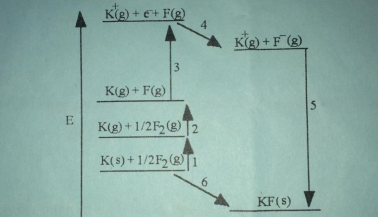# Problem: The diagram below is the Born-Haber cycle for the formation of crystalline potassium fluoride KF. Which energy change (by number) corresponds to the lattice energy of KF? a. 5 b. 2 c. 4 d. 1 e. 6

###### Problem Details

The diagram below is the Born-Haber cycle for the formation of crystalline potassium fluoride KF.

Which energy change (by number) corresponds to the lattice energy of KF?

a. 5

b. 2

c. 4

d. 1

e. 6What scientific concept do you need to know in order to solve this problem?

Our tutors have indicated that to solve this problem you will need to apply the Born Haber Cycle concept. You can view video lessons to learn Born Haber Cycle. Or if you need more Born Haber Cycle practice, you can also practice Born Haber Cycle practice problems.

What is the difficulty of this problem?

Our tutors rated the difficulty ofThe diagram below is the Born-Haber cycle for the formation ...as medium difficulty.

How long does this problem take to solve?

Our expert Chemistry tutor, Jules took 1 minute and 28 seconds to solve this problem. You can follow their steps in the video explanation above.

What professor is this problem relevant for?

Based on our data, we think this problem is relevant for Professor Harman's class at UCR.